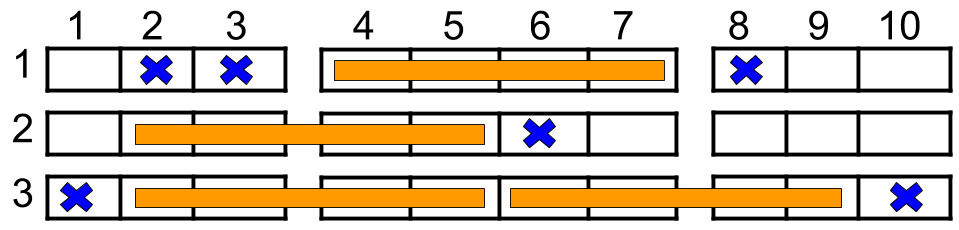1386. Cinema Seat AllocationA cinema has `n` rows of seats, numbered from 1 to `n` and there are ten seats in each row, labelled from 1 to 10 as shown in the figure above.

Given the array `reservedSeats` containing the numbers of seats already reserved, for example, `reservedSeats[i] = [3,8]` means the seat located in row 3 and labelled with 8 is already reserved.

Return the maximum number of four-person groups you can assign on the cinema seats. A four-person group occupies four adjacent seats in one single row. Seats across an aisle (such as [3,3] and [3,4]) are not considered to be adjacent, but there is an exceptional case on which an aisle split a four-person group, in that case, the aisle split a four-person group in the middle, which means to have two people on each side.

Example 1:```Input: n = 3, reservedSeats = [[1,2],[1,3],[1,8],[2,6],[3,1],[3,10]]
Output: 4
Explanation: The figure above shows the optimal allocation for four groups, where seats mark with blue are already reserved and contiguous seats mark with orange are for one group.
```

Example 2:

```Input: n = 2, reservedSeats = [[2,1],[1,8],[2,6]]
Output: 2
```

Example 3:

```Input: n = 4, reservedSeats = [[4,3],[1,4],[4,6],[1,7]]
Output: 4
```

Constraints:

• `1 <= n <= 10^9`
• `1 <= reservedSeats.length <= min(10*n, 10^4)`
• `reservedSeats[i].length == 2`
• `1 <= reservedSeats[i] <= n`
• `1 <= reservedSeats[i] <= 10`
• All `reservedSeats[i]` are distinct.

1386. Cinema Seat Allocation
``````#![allow(clippy::unreadable_literal)]
struct Solution;
use std::collections::HashMap;

impl Solution {
fn max_number_of_families(n: i32, reserved_seats: Vec<Vec<i32>>) -> i32 {
let n = n as usize;
let mut hm: HashMap<usize, u16> = HashMap::new();
for seat in reserved_seats {
let i = (seat - 1) as usize;
let j = (seat - 1) as usize;
*hm.entry(i).or_default() |= 1 << j;
}
let mut res = 0;
for &row_bitset in hm.values() {
res += Self::num_of_families(row_bitset);
}
res += (n - hm.len()) * 2;
res as i32
}

fn num_of_families(row_bitset: u16) -> usize {
let two = 0b0111111110;
let mid = 0b0001111000;
let left = 0b0111100000;
let right = 0b0000011110;
if row_bitset & two == 0 {
2
} else {
if row_bitset & mid == 0 {
1
} else {
if row_bitset & left == 0 || row_bitset & right == 0 {
1
} else {
0
}
}
}
}
}

#[test]
fn test() {
let n = 3;
let reserved_seats = vec_vec_i32![[1, 2], [1, 3], [1, 8], [2, 6], [3, 1], [3, 10]];
let res = 4;
assert_eq!(Solution::max_number_of_families(n, reserved_seats), res);
let n = 2;
let reserved_seats = vec_vec_i32![[2, 1], [1, 8], [2, 6]];
let res = 2;
assert_eq!(Solution::max_number_of_families(n, reserved_seats), res);
let n = 4;
let reserved_seats = vec_vec_i32![[4, 3], [1, 4], [4, 6], [1, 7]];
let res = 4;
assert_eq!(Solution::max_number_of_families(n, reserved_seats), res);
}
``````